# Ultra Binary Calc

100+Everyone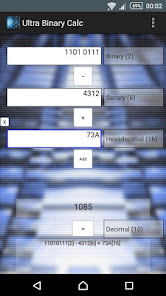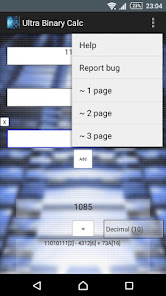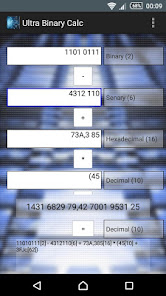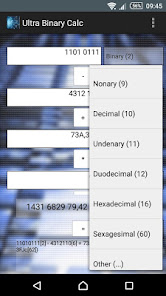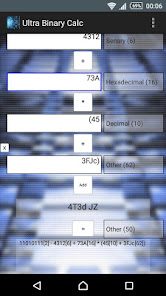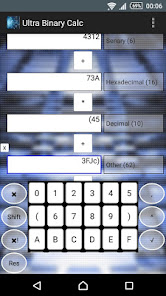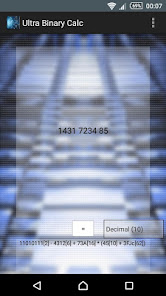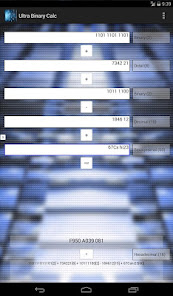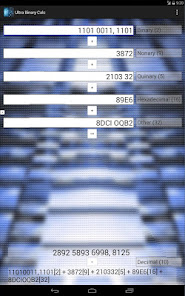It's a calculator, in which you can do calculations in many different numerical systems (from 2 to 62 numerical system). You can among others:

- sum
- subtraction
- multiplication
- division
- exponentiation
- square root
- calculations on parentheses
- calculations on floating point numbers

and all numbers and result may to be in others numerical systems.
Updated on
Jul 26, 2015

## Data safety